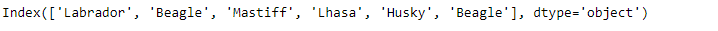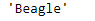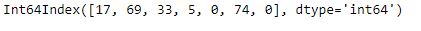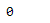Related Articles
Python | Pandas Index.min()
• Last Updated : 17 Dec, 2018

Python is a great language for doing data analysis, primarily because of the fantastic ecosystem of data-centric python packages. Pandas is one of those packages and makes importing and analyzing data much easier.

Pandas` Index.min()` function returns the minimum value of the Index. The function works with both numerical as well as the string type objects. In case of string type object it returns the string which has smallest value in lexicographical order.

Syntax: Index.min()

Parameters : Doesn’t take any parameter.

Returns : scalar: Minimum value.

Example #1: Use `Index.min()` function to find the minimum element in the given Index.

 `# importing pandas as pd``import` `pandas as pd`` ` `# Creating the Index``idx ``=` `pd.Index([``'Labrador'``, ``'Beagle'``, ``'Mastiff'``, ``'Lhasa'``, ``'Husky'``, ``'Beagle'``])`` ` `# Print the Index``idx`

Output :Now we find the min value in the given Index.

 `# return min value.``idx.``min``()`

Output :As we can see in the output, the function has returned ‘Beagle’ which has the smallest lexicographical order among the values present in the Index.

Example #2: Use `Index.min()` function to find the minimum value in the Index.

 `# importing pandas as pd``import` `pandas as pd`` ` `# Creating the Index``idx ``=` `pd.Index([``17``, ``69``, ``33``, ``5``, ``0``, ``74``, ``0``])`` ` `# Print the Datetime Index``idx`

Output :Now we will find the minimum value among the labels of the Index.

 `# the function will return the minimum``#  value present in the Index``idx.``min``()`

Output :As we can see in the output, the function has returned 0 which is the smallest value among the labels of the Index.

Attention geek! Strengthen your foundations with the Python Programming Foundation Course and learn the basics.

To begin with, your interview preparations Enhance your Data Structures concepts with the Python DS Course.

My Personal Notes arrow_drop_up